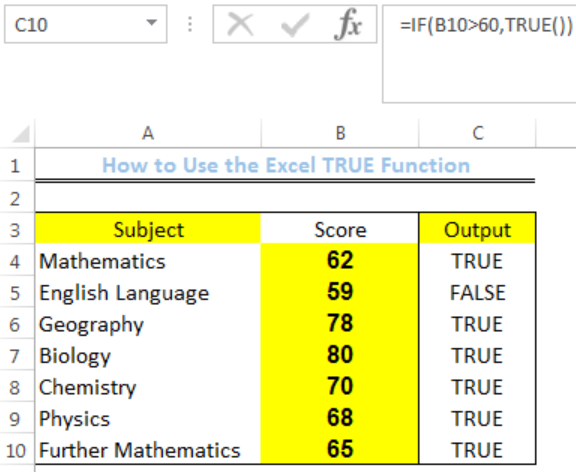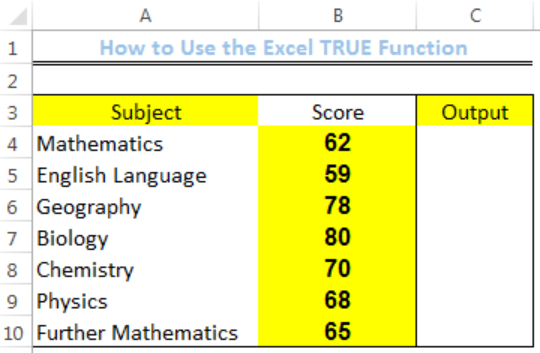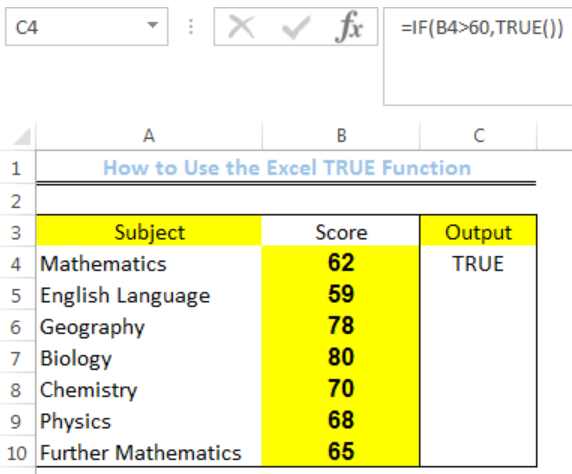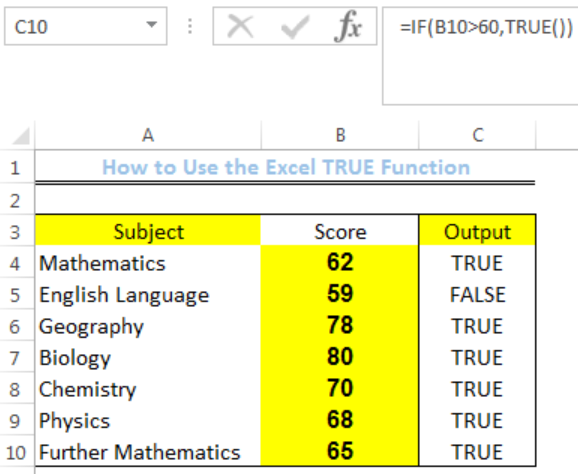Get instant live expert help with Excel or Google Sheets“My Excelchat expert helped me in less than 20 minutes, saving me what would have been 5 hours of work!”

#### Post your problem and you’ll get expert help in seconds.

Your message must be at least 40 characters
Our professional experts are available now. Your privacy is guaranteed.

# How to Use the Excel TRUE Function

We can use the TRUE function to return a result if the criteria is satisfied. The steps below will walk through the process.Figure 1: How to Use the TRUE Function

## Syntax

`=TRUE()`

## Formula

`=IF(B4>60,TRUE())`

## Setting up the Data

• We will set up the data by inputting the SUBJECTS into Column A
• Column B will contain the scores for each subject
• Column C is where we want the formula to return the result for the TRUE FunctionFigure 2: Setting up the Data

## Using the True Function

• We will click on Cell C4
• We will insert the formula below into the cell
`=`
`IF(B4>60,TRUE())`
• We will press the enter keyFigure 3: Output of the TRUE function for Cell B4

• We will click on Cell C4 again
• We will double-click on the fill handle (the small plus sign at the bottom right of Cell C4) and drag down to copy the formula into the other cellsFigure 4: Result of the TRUE Function

## Explanation

The value in the cell is checked if it is greater than 60. If it is, the IF Function returns TRUE and FALSE if otherwise.

## Note

If we enter logical expressions such as =B4>60 into Cell C4, we will still get the same return value of TRUE.

## Instant Connection to an Expert through our Excelchat Service

Most of the time, the problem you will need to solve will be more complex than a simple application of a formula or function. If you want to save hours of research and frustration, try our live Excelchat service! Our Excel Experts are available 24/7 to answer any Excel question you may have. We guarantee a connection within 30 seconds and a customized solution within 20 minutes.

### Did this post not answer your question? Get a solution from connecting with the expert.Another blog reader asked this question today on Excelchat:
Solution examplesCould you help me by explaining the IF function?
Solved by G. L. in 24 minsI have a question about Conditional Formatting. =IF('Sheet1'!A1="X",IF('Sheet1'!B1="Y", TRUE, FALSE),FALSE) the set the cell background to red. I would like to make it so that if Sheet1'!B1="Z" I set the back to blue. How can I achieve this.
Solved by B. F. in 39 minsI need to find out if the 2018 yearly sales goals were met if the yearly sales were \$25,000 or more using an IF logical function and to set the formula to return a value of YES if met, and NO if not
Solved by A. A. in 18 minsI filtered data in column A (it is labeled ID #) of a data set. On my next worksheet, column A is also the ID #, but it is a different data set. I want to filter the ID #'s the same for the two sheets, but how do I do that being that I am working with two different sets of data?
Solved by V. H. in 22 minsi want to create a very simple if function in a cell which is, if one cell is less than 1250, then the automatic value in the current cell is 250, dead easy, for someone who knows! Thank you.
Solved by A. H. in 14 mins## Subscribe to Excelchat.coAnother blog reader asked this question today on Excelchat: